# 修改折线图坐标 | Python 数据可视化库 Matplotlib 快速入门之八

## 完善原始折线图（辅助显示层）

### 准备数据并画出初始折线图

1. 准备数据x、y
2. 创建画布
3. 绘制图像
4. 显示图像
import random

x = range(60)
y_shanghai  = [random.uniform(15, 18) for i in x]

plt.figure(figsize=(20, 8), dpi=80)
plt.plot(x, y_shanghai)
plt.show()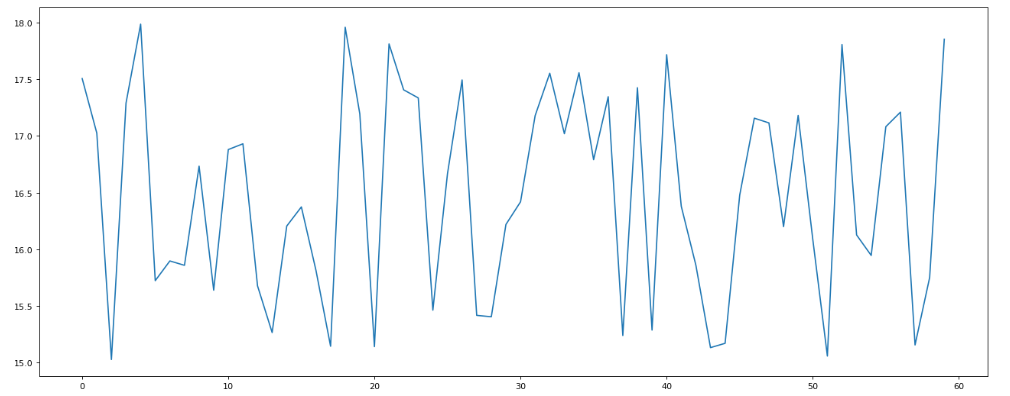### 添加自定义x，y刻度

• plt.xticks(x, **kwargs)
x：要显示的刻度值
• plt.yticks(y, **kwargs)

y：要显示的刻度值


plt.xticks(x[::5])
#plt.yticks(range(40), [::5])
plt.yticks(range(0, 40, 5))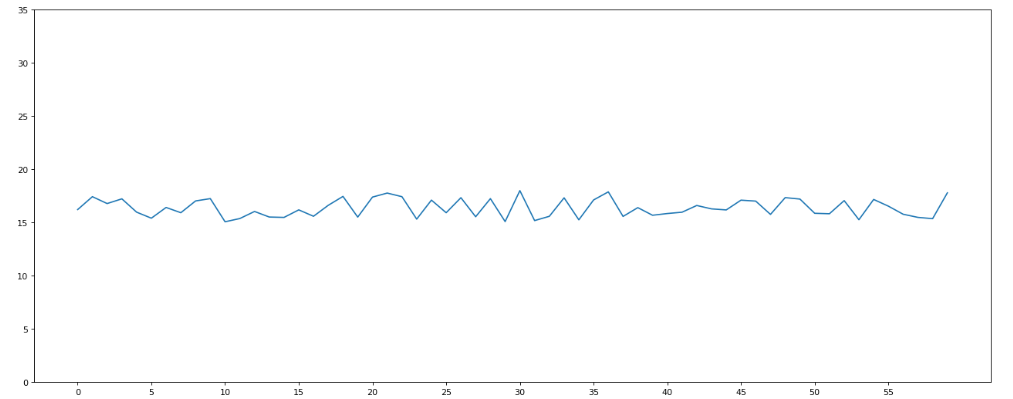x_lable = ["11点{}分".format(i) for i in x]
plt.xticks(x[::5], x_lable[::5])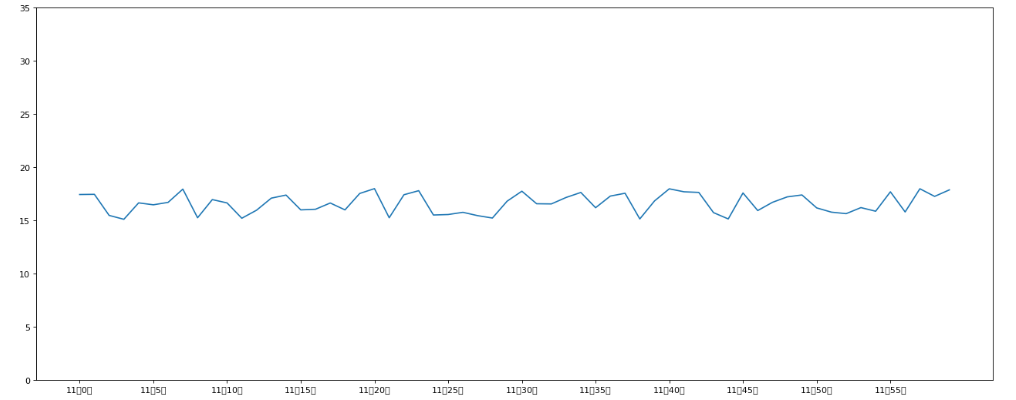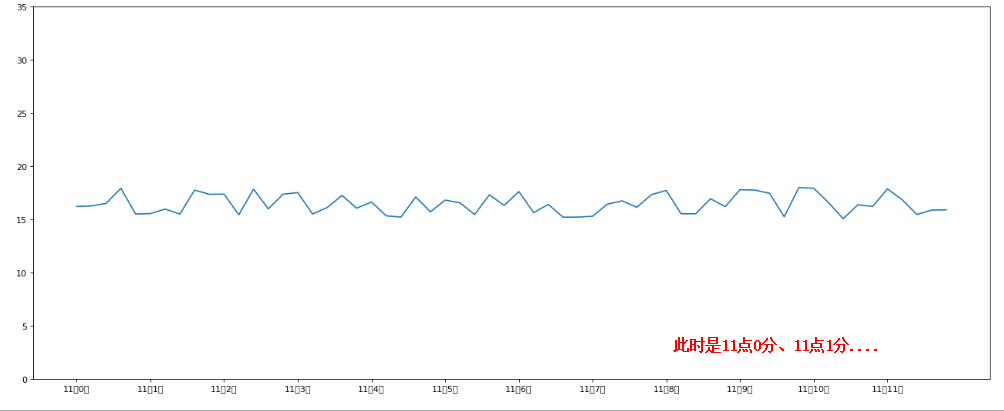### 配套视频课程，点击这里查看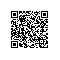Python学习站使用钉钉扫一扫加入圈子
+ 订阅

Python学习资料大全，包含Python编程学习、实战案例分享、开发者必知词条等内容。